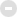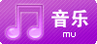##用户名 Email 自动登录 找回密码 密码 中文注册

## day = new Date( ) wz_Hour = day.getHours( ) var wz_warmprompt = ""; if (wz_Hour == 0) wz_warmprompt = "现在已经过凌晨了，身体是无价的资本喔，早点休息吧！" if (wz_Hour == 1) wz_warmprompt = "凌晨1点多了，工作是永远都做不完的，别熬坏身子！" if (wz_Hour == 2) wz_warmprompt = "该休息了，身体可是革命的本钱啊！" if (wz_Hour == 3) wz_warmprompt = "夜深了，熬夜很容易导致身体内分泌失调，长痘痘的！" if (wz_Hour == 4) wz_warmprompt = "四点过了，你明天不上班？？？" if (wz_Hour == 5) wz_warmprompt = "你知道吗，此时是国内网络速度最快的时候！" if (wz_Hour == 6) wz_warmprompt = "清晨好，这麽早就上论坛啦，昨晚做的梦好吗？ " if (wz_Hour == 7) wz_warmprompt = "新的一天又开始了，祝你过得快乐!" if (wz_Hour == 8) wz_warmprompt = "早上好，一天之际在于晨，又是美好的一天！" if ((wz_Hour == 9) || (wz_Hour ==10)) wz_warmprompt = "上午好！今天你看上去好精神哦！" if (( wz_Hour == 11) || (wz_Hour == 12)) wz_warmprompt = "该吃午饭啦！有什么好吃的？您有中午休息的好习惯吗？" if (( wz_Hour >= 13) && (wz_Hour <= 17)) wz_warmprompt = "下午好！外面的天气好吗？记得朵朵白云曾捎来朋友殷殷的祝福。" if (( wz_Hour >= 17) && (wz_Hour <= 18)) wz_warmprompt = "太阳落山了！快看看夕阳吧！如果外面下雨，就不必了 ^_^" if (( wz_Hour >= 18) && (wz_Hour <= 19)) wz_warmprompt = "晚上好，今天的心情怎么样，来论坛和我们诉说吧！" if (( wz_Hour >= 19) && (wz_Hour <= 21)) wz_warmprompt = "忙碌了一天，累了吧？来玩玩应用游戏，放松下吧！" if (( wz_Hour >= 22) && (wz_Hour <= 23)) wz_warmprompt = "这么晚了，还在上网？早点洗洗睡吧，睡前记得洗洗脸喔！" document.write("　　<font color='#2E9AFE'>『温馨提示』") document.write(wz_warmprompt) document.write("</font>")

### 热门主题分区版主: 龙行天下, tiantian

## 『天籁之音』## 『高清MV版块』 (1)

103 / 87569 / 391